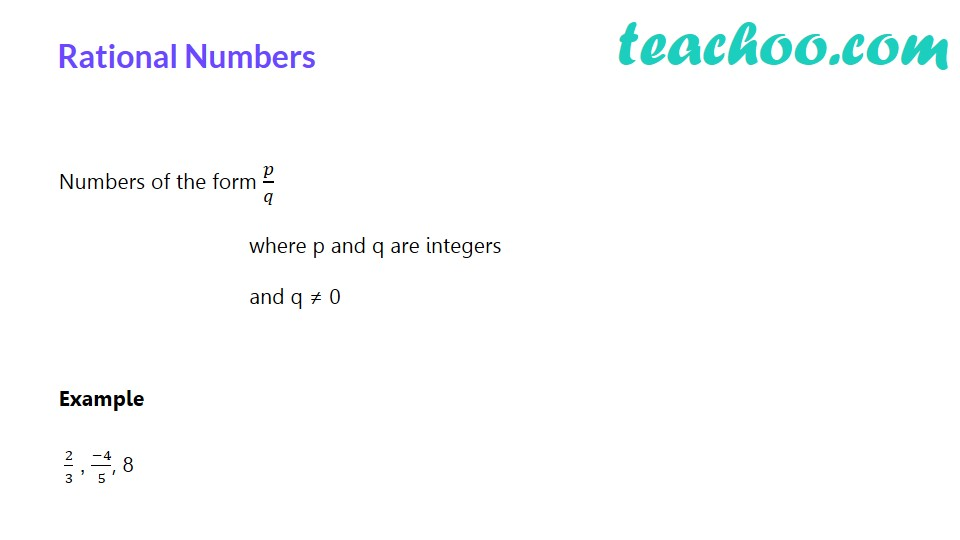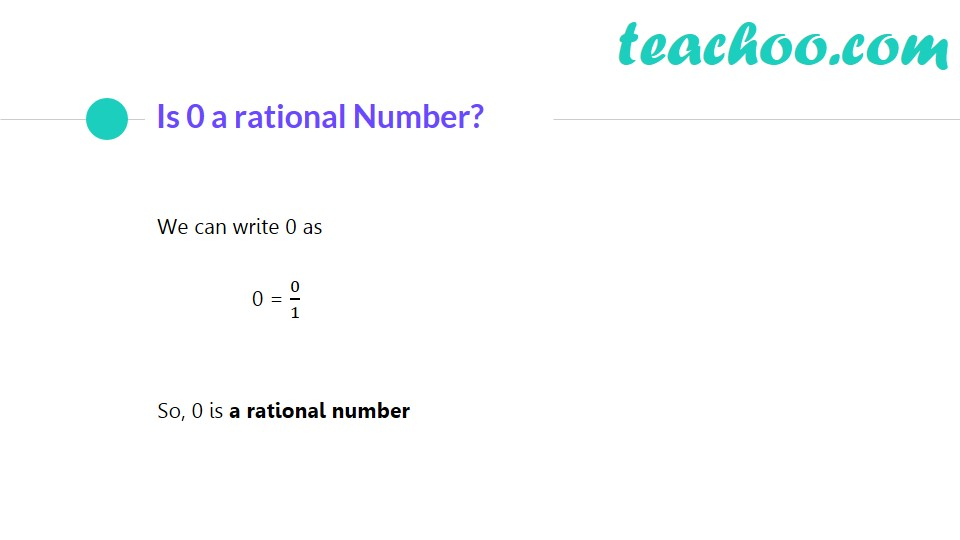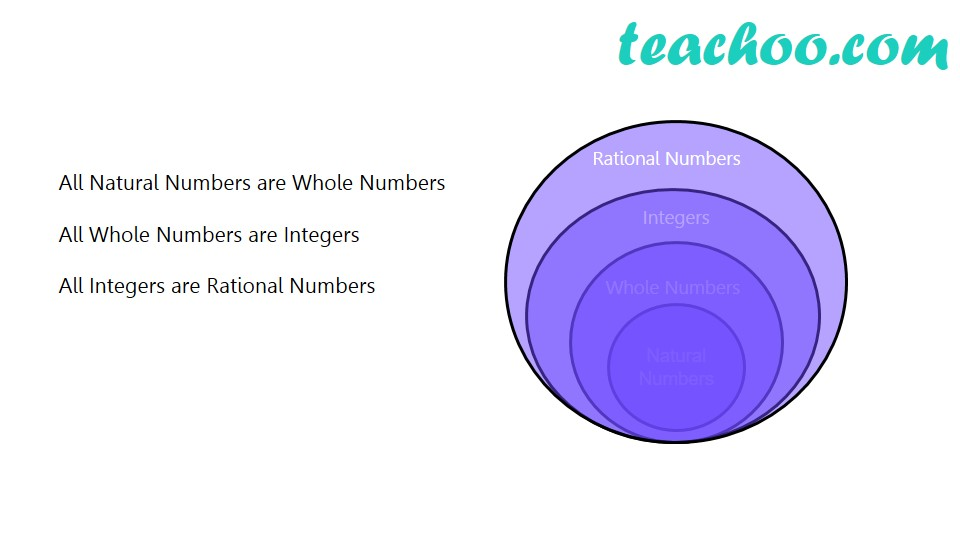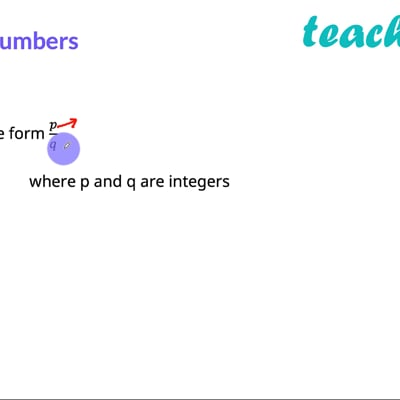Types of Numbers

Chapter 1 Class 8 Rational Numbers
Concept wiseThis video is only available for Teachoo black users

Rational numbers are numbers which can be made by dividing two integers.

Example:

If we divide 1 by 2,

we get 1/2

Which is a rational number

Similarly,

If we divide 2 by 3,

We get 2/3

Which is a rational number

Similarly, rational numbers can be

3/8, 4/6, 9/21 and son on

#### Is 2 a rational number?

2 can be written

as 2 = 2/1

Since we have integers on numerator as well as denominator,

So, it is a rational number

#### Is (-4)/9 a rational number?

Since (-4)/9 has integers on numerator as well as denominator,

So, it is a rational number

#### Is –31 a rational number?

–31 can be written

as –31 = (-31)/1

Since we have integers on numerator as well as denominator,

So, it is a rational number

And with 0?

Let’s see

#### Is 0/8 a rational number?

Since we have integers on numerator as well as denominator,

So, it is a rational number

#### Is 5/0 a rational number?

We cannot have 0 in the denominator

So, 5/0 is not a rational number

It is actually not defined.

### To mathematically define Rational numbers

We say that Rational numbers are numbers in the form p/q, where p & q are integers, and q ≠ 0

#### But what about Natural Numbers, Whole Numbers and Integers?

We know that

Natural Numbers − Numbers starting from 1

Eg: 1, 2, 3, 4, 5……..

Whole Numbers − Numbers starting from 0

0, 1, 2, 3, 4, 5……..

Integers − Integers are both positive & negative numbers & zero

….. −3, −2, −1, 0, 1, 2, 3,…..

Now,

2 = 2/1

So,  it is in p/q form

∴ It is rational number

So, all natural numbers are rational numbers

Similarly,

0 = 0/1

So,  it is in p/q form

∴ It is rational number

So, all whole numbers are rational numbers

And,

–3 = (-3)/1

So,  it is in p/q form

∴ It is rational number

So, all integers are rational numbers

∴ All natural numbers, whole numbers, integers are rational numbers

Introducing your new favourite teacher - Teachoo Black, at only ₹83 per month

### Transcript

Rational Numbers Numbers of the form 𝑝/𝑞 where p and q are integers and q ≠ 0 Example 2/3 , (−4)/5, 8 Is 0 a rational Number? We can write 0 as 0 = 0/1 So, 0 is a rational number All Natural Numbers are Whole Numbers All Whole Numbers are Integers All Integers are Rational Numbers